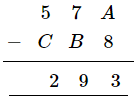# Replace A, B, C by suitable numerals:

Question:

Replace ABC by suitable numerals:Solution:

$A-8=3$

​This implies that 1 is borrowed.

$11-8=3$

$\Rightarrow A=1$

Then, $7-B=9$

1 is borrowed from 7.

$\therefore 16-B=9$

$\quad \Rightarrow B=7$

Further, $5-C=2$

But 1 has been borrowed from $5 .$

$\therefore 4-C=2$

$\Rightarrow C=2$

$\therefore A=1, B=7$ and $C=2$